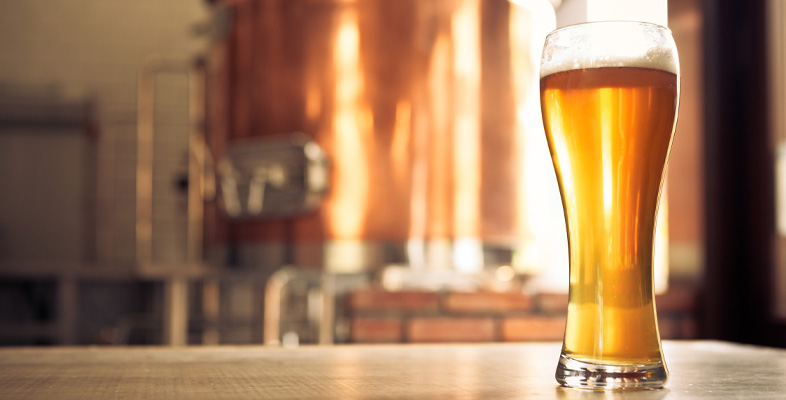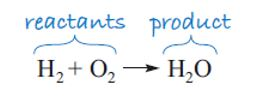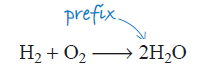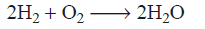Science, Maths & Technology

### Become an OU studentThe science of alcohol

Start this free course now. Just create an account and sign in. Enrol and complete the course for a free statement of participation or digital badge if available.

# 2.2 Chemical reactions of ethanol

When something happens to a chemical compound and a change of some sort takes place, there has been a chemical reaction. Ethanol undergoes a number of important chemical reactions – you will come across several of them as you work through this course. But this week you’ll start by looking at two chemical reactions in particular:

• the combustion of ethanol
• how it reacts to form acetic acid, the main component in vinegar.

But first, you need to see how chemists express a chemical reaction in the form of a chemical equation.

In writing a chemical equation you need to state what you start with and what you end up with. Let’s look at a simple example using two elements: hydrogen gas, H2, and oxygen gas, O2, which react with each other (explosively) to form water, H2O, and nothing else.

The simplest way of writing this down chemically is to note the formulae of the elements that react with each other – the reactants – and use an arrow to indicate what has been formed in the reaction – the product, as shown in Figure 4.Figure 4 The formation of water

Notice the convention of reactants on the left and products on the right. This equation is useful in that it tells you, in a shorthand form, which types of molecule you start with and finish with, and you can see that the reactant molecules interact in some way. However, if you look closely at the equation, you’ll see that there is a problem in that the number of atoms in the reactants is not equal to the number of atoms in the product, and atoms cannot be destroyed (or created) in a chemical reaction.

Chemists would say that this equation is ‘not balanced’. It is important to be able to produce a balanced equation for a chemical reaction, because it is only from this that the quantities of the substances involved can be calculated, and hence the energy absorbed or released by a reaction can be determined. Our ultimate goal is to calculate the energy involved in the combustion of ethanol. So we need to find a way to balance the equation. Let’s start with the first element, hydrogen. Looking at Figure 4, you can see that the hydrogen atoms balance – there are two on either side of the arrow. Moving to oxygen, there are two oxygen atoms on the left, but at present only one on the right.

As an atom of oxygen cannot be lost, and only water has been produced, two molecules of water must have been made. To indicate this, a prefix ‘2’ is placed in front of the formula for the water molecule (Figure 5).Figure 5 Balancing the equation for the formation of water

Unfortunately, this immediately unbalances the equation again as now there are two hydrogen atoms on the left, and four (2 × 2) on the right, and this is where you must hold your nerve – you simply go through the process again! This time, using two reactant molecules of hydrogen corrects the problem (Figure 6):Figure 6 A balanced equation for the formation of water

And now the equation ‘balances’.

Notice again the different uses of numbers in the equation. The subscript 2 in the formula indicates that two hydrogen atoms are contained in the water molecule. The prefix 2 in front of the water formula indicates that there are two H2O molecules.